# A 3rd Grade Math Lesson on Finding the Area of Rectangles Using Multiplication

Page content

Without overlapping or leaving gaps, cover the rectangular figures with “unit squares”. To determine the areas of geometric figures, count the number of “unit squares” used to cover the figures. Record the lengths and widths of the rectangular figures, multiply the lengths and the widths, compare the products to the areas.

Lesson Objective: The lesson is aligned to the Common Core State Standards for Mathematics – 3.MD.7a Geometric Measurement – Relate area to the operations of multiplication.

Materials Required: unit square, graph paper, calculator

## Lesson Procedure:

Identifying Area of a “Unit Square” as One Square Centimeter

1. Identify the side length of the “tile” on the graph paper as one unit.

2. Identify the area of the “tile” as one square unit.3. Count the number of “tiles” shaded in Figure A.

4. How many “tiles” are shaded in Figure A?

5. What is the area, in square units, of Figure A?

6. What is the unit length of Figure A?

7. What is the unit width of Figure A?

8. Multiply the length times the width of Figure A.

9. Compare the number of tiles used to cover Figure A to the product of the length and width of Figure A.

10. Write a statement describing the comparison.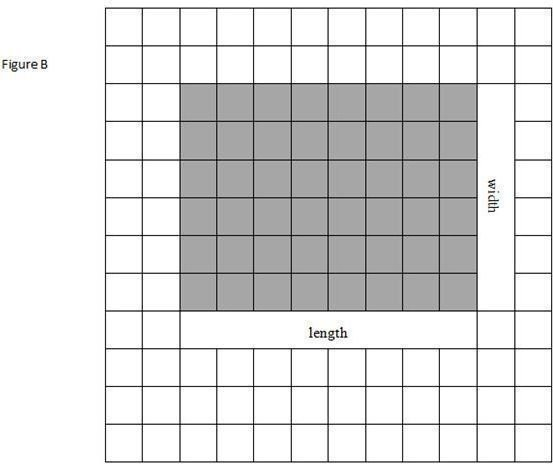11. Count the number of “tiles” shaded in Figure B.

12. How many “tiles” are shaded in Figure B?

13. What is the area, in square units, of Figure B?

14. What is the unit length of Figure B?

15. What is the unit width of Figure B?

16. Multiply the length times the width of Figure B.

17. Compare the number of tiles used to cover Figure B to the product of the length and width of Figure B.

18. Write a statement describing the comparison.

4. 63

5. 63 square units

6. 9 units

7. 7 units

10. The number of tiles used to cover Figure A is 63. The product of the length, 9, and the width, 7, is 63. The number of tiles used to cover Figure A is the same as the product of the length and the width of Figure A. The area of Figure A is 63 square units.

12. 48

13. 48 square units

14. 8 units

15. 6 units

18. The number of tiles used to cover Figure B is 48. The product of the length, 8, and the width, 6, is 48. The number of tiles used to cover Figure B is the same as the product of the length and the width of Figure B. The area of Figure B is 48 square units.

## Individual or Group Work: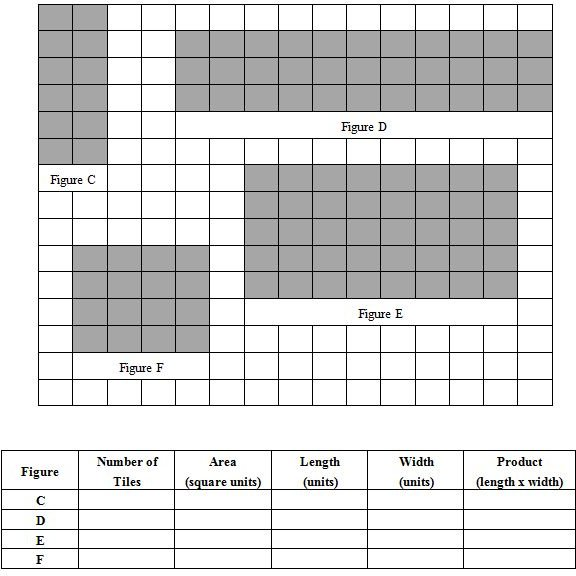Complete the chart:

1. Record the number of tiles shaded in Figures C, D, E, and F.

2. Record the area, in square units, of Figures C, D, E, and F.

3. Record the lengths and the widths of Figures C, D, E, and F.

4. Record the products of the lengths and the widths of Figures C, D, E, and F.5. Write a statement to compare the number of tiles used to cover the figures to the products of the lengths and widths of the figures.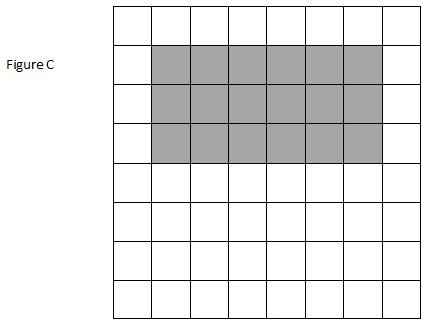6. The numbers of tiles used to cover the figures is equal to the products of the lengths and the widths of the figures.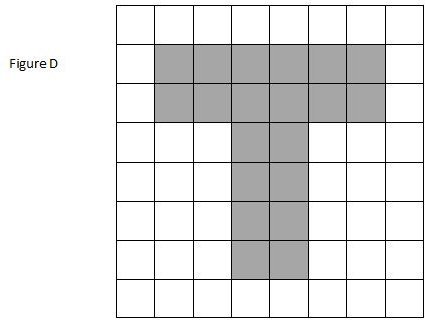7. Count the number of “unit squares” shaded in Figure D.

8. How many “unit squares” are shaded in Figure D?

9. What is the area, in square centimeters, of Figure D?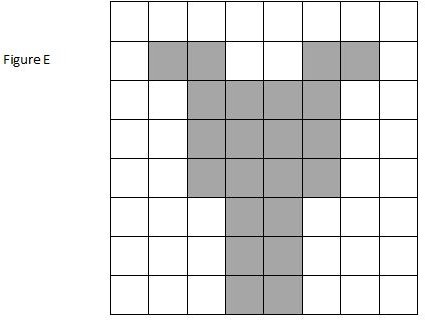10. Count the number of “unit squares” shaded in Figure E.

11. How many “unit squares” are shaded in Figure E?

12. What is the area, in square centimeters, of Figure E?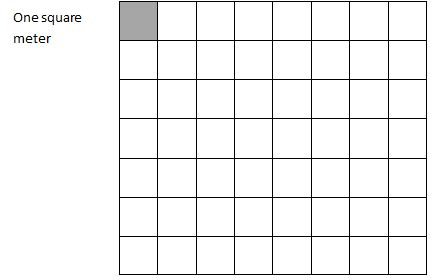Identifying Area of a “Unit Square” as One Square Meter

13. Identify the side length of the “unit square” on the graph paper as one meter, m.

14. Identify the area of the “unit square” as one square meter.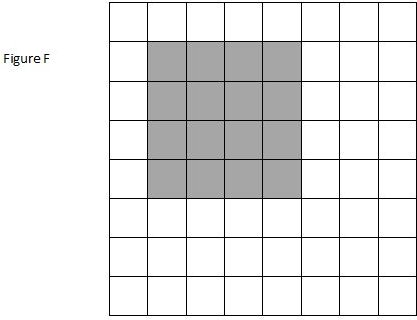15. Count the number of “unit squares” shaded in Figure F.

16. How many “unit squares” are shaded in Figure F?

17. What is the area, in square meters, of Figure F?

4. 25

5. 25 square centimeters

7. 18

8. 18 square centimeters

10. 20

11. 20 square centimeters

13. 22

14. 22 square centimeters

18. 16

19. 16 square centimeters

## Individual or Group Work: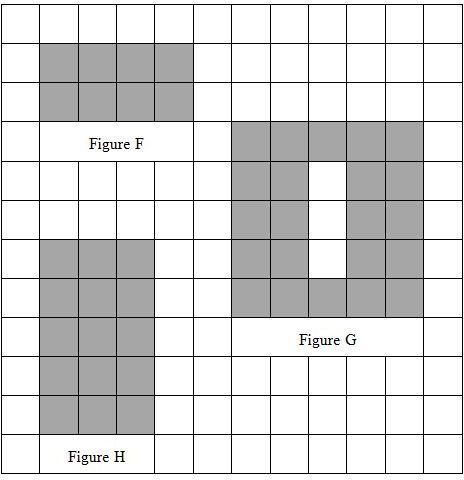1. The area of each “unit square” measures one square meter, sq m.

2. Count the number of “unit squares” shaded in Figures F, G, and H.

3. How many “unit squares” are shaded in Figures F, G, and H?

4. What is the area, in square meters, of Figures F, G, and H?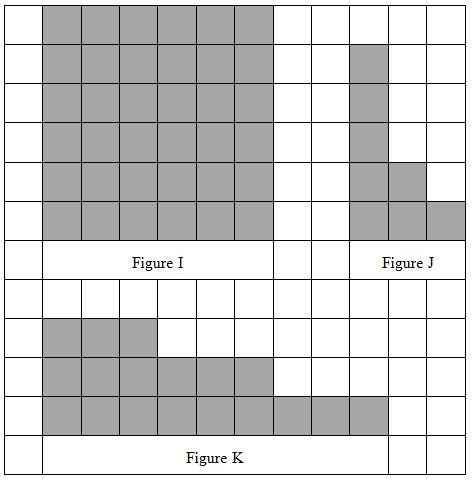5. The area of each “unit square” measures one square inch, sq in.

6. Count the number of “unit squares” shaded in Figures I, J, and K.

7. How many “unit squares” are shaded in Figures I, J, and K?

8. What is the area, in square inches, of Figures I, J, and K?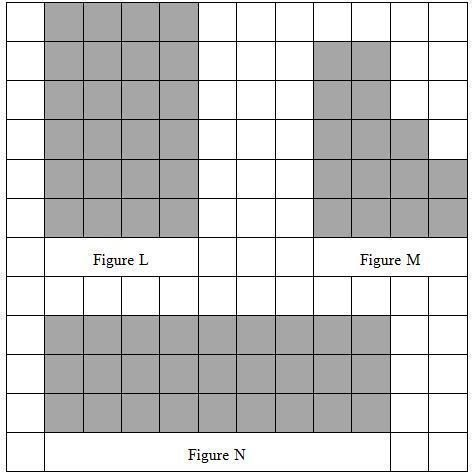9. The area of each “unit square” measures one square foot, sq ft.

10. Count the number of “unit squares” shaded in Figures L, M, and N.

11. How many “unit squares” are shaded in Figures L, M, and N?

12. What is the area, in square feet, of Figures L, M, and N?

3. 8, 22, 15
4. 8 square meters, 22 square meters, 15 square meters
7. 36, 8, 18
8. 36 square inches, 8 square inches, 18 square inches
11. 24, 15, 27
12. 24 square feet, 15 square feet, 27 square feet